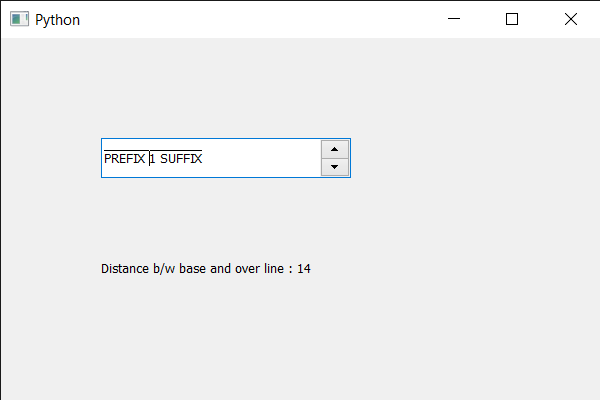Related Articles
PyQt5 QSpinBox – Getting over line position
• Last Updated : 28 May, 2020

In this article we will see how we can get over line position of the spin box’s text. Over line position is the distance from the base line to where an over line should be drawn. An over line, overs core, or over bar, is a typographical feature of a horizontal line drawn immediately above the text. In mathematical notation, an over line has been used for a long time as a vinculum, a way of showing that certain symbols belong together.

In order to do this we use `overlinePos` method with the spin box’s QFontMetrics object.

Syntax : font_metrics.overlinePos()

Argument : It takes no argument

Return : It returns integer.

Below is the implementation

 `# importing libraries``from` `PyQt5.QtWidgets ``import` `*` `from` `PyQt5 ``import` `QtCore, QtGui``from` `PyQt5.QtGui ``import` `*` `from` `PyQt5.QtCore ``import` `*` `import` `sys`` ` ` ` `class` `Window(QMainWindow):`` ` `    ``def` `__init__(``self``):``        ``super``().__init__()`` ` `        ``# setting title``        ``self``.setWindowTitle(``"Python "``)`` ` `        ``# setting geometry``        ``self``.setGeometry(``100``, ``100``, ``600``, ``400``)`` ` `        ``# calling method``        ``self``.UiComponents()`` ` `        ``# showing all the widgets``        ``self``.show()`` ` `        ``# method for widgets``    ``def` `UiComponents(``self``):``        ``# creating spin box``        ``self``.spin ``=` `QSpinBox(``self``)`` ` `        ``# setting geometry to spin box``        ``self``.spin.setGeometry(``100``, ``100``, ``250``, ``40``)`` ` `        ``# setting range to the spin box``        ``self``.spin.setRange(``1``, ``999999``)`` ` `        ``# setting prefix to spin``        ``self``.spin.setPrefix(``"PREFIX "``)`` ` `        ``# setting suffix to spin``        ``self``.spin.setSuffix(``" SUFFIX"``)`` ` `        ``# setting over line to the font``        ``font ``=` `self``.spin.font()``        ``font.setOverline(``True``)``        ``self``.spin.setFont(font)`` ` `        ``# creating a label``        ``label ``=` `QLabel(``self``)`` ` `        ``# making label multi line``        ``label.setWordWrap(``True``)`` ` `        ``# setting geometry to the label``        ``label.setGeometry(``100``, ``200``, ``300``, ``60``)`` ` `        ``# getting font metrics``        ``f_metrics ``=` `self``.spin.fontMetrics()`` ` `        ``# getting over line position``        ``value ``=` `f_metrics.overlinePos()`` ` `        ``# setting text to the label``        ``label.setText(``"Distance b / w base and over line : "` `+` `str``(value))`` ` ` ` `# create pyqt5 app``App ``=` `QApplication(sys.argv)`` ` `# create the instance of our Window``window ``=` `Window()`` ` `# start the app``sys.exit(App.``exec``())`

Output :Attention geek! Strengthen your foundations with the Python Programming Foundation Course and learn the basics.

To begin with, your interview preparations Enhance your Data Structures concepts with the Python DS Course.

My Personal Notes arrow_drop_up